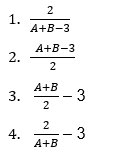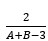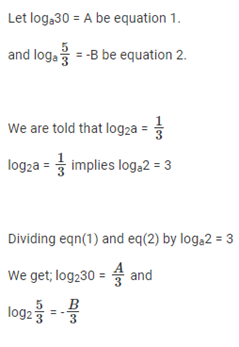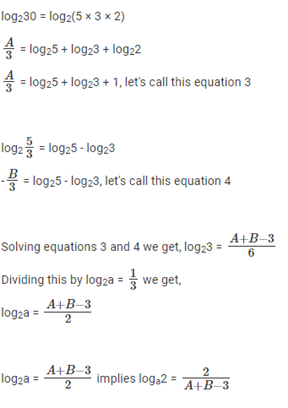# If log (base a)30 = A - Quantitative Aptitude - CAT Logarithm

## CAT 2020 - Slot 3 - Quantitative Aptitude - logarithm - Question 3 - If log (base a)30 = A

Q. 3: If log (base a)30 = A log(base a)(5/3)= -B and log(base 2)a=1/3, then log(base 3)a equals ?Correct Answer:(Option 1)### Past Year Question Paper & SolutionsCounselling Session
By IIM Mentor

#### Free Material Area